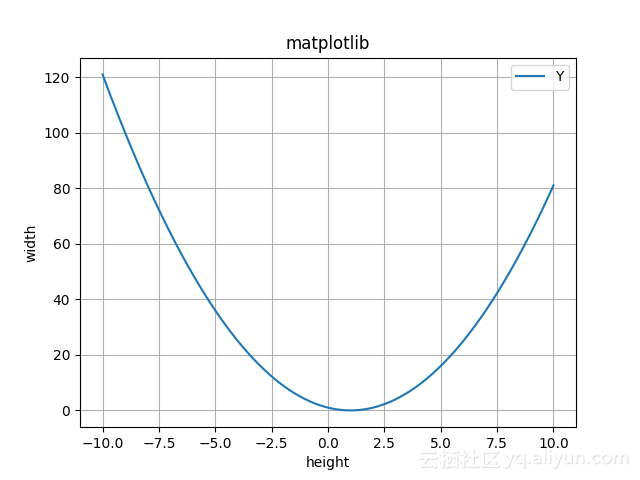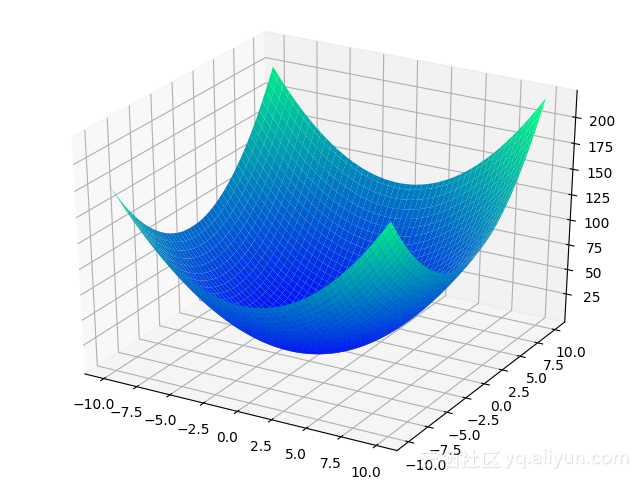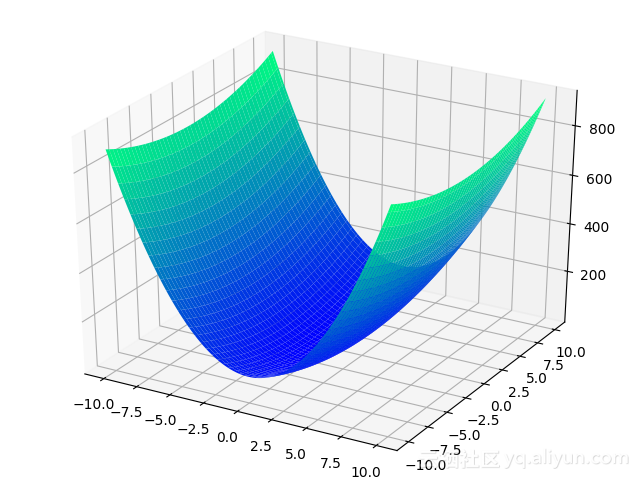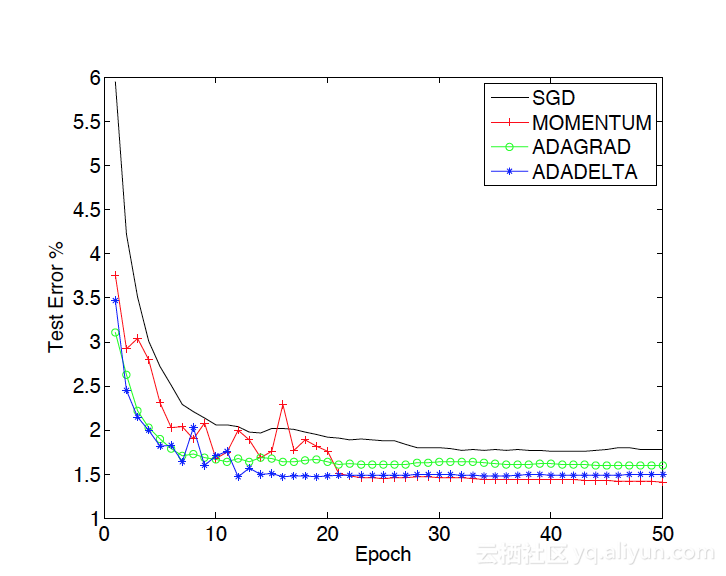#Tensorflow快餐教程(7) - 梯度下降

0
0
01. 云栖社区>
2. 博客>
3. 正文

## Tensorflow快餐教程(7) - 梯度下降

lusing 2018-05-03 11:55:02 浏览1948

# 梯度下降

import tensorflow as tf
import numpy as np

trX = np.linspace(-1, 1, 101)
trY = 2 * trX + np.random.randn(*trX.shape) * 0.33 # 创建一些线性值附近的随机值

X = tf.placeholder("float")
Y = tf.placeholder("float")

def model(X, w):
return tf.multiply(X, w) # X*w线性求值，非常简单

w = tf.Variable(0.0, name="weights")
y_model = model(X, w)

cost = tf.square(Y - y_model) # 用平方误差做为优化目标

train_op = tf.train.GradientDescentOptimizer(0.01).minimize(cost) # 梯度下降优化

# 开始创建Session干活！
with tf.Session() as sess:
# 首先需要初始化全局变量，这是Tensorflow的要求
tf.global_variables_initializer().run()

for i in range(100):
for (x, y) in zip(trX, trY):
sess.run(train_op, feed_dict={X: x, Y: y})

print(sess.run(w)) 

train_op = tf.train.GradientDescentOptimizer(0.01).minimize(cost)

## 从画函数图形说起

import matplotlib.pyplot as plt
import numpy as np

x = np.linspace(-10,10,1000)
y = x ** 2 - 2 * x+ 1
plt.plot(x, y)
plt.title("matplotlib")
plt.xlabel("height")
plt.ylabel("width")
# 设置图例
plt.legend(["X","Y"], loc="upper right")
plt.grid(True)
plt.show()## 求函数的最小值

$x_{n+1}=x_n - \eta\frac{df(x)}{dx}$

$f(x)=x^2-2x+1$的导函数为$f^{'}(x)=2x-2$

## 晋阶三维世界

cost = tf.square(Y - y_model)

from mpl_toolkits.mplot3d import Axes3D
import numpy as np
import matplotlib.pyplot as plt

# 创建 3D 图形对象
fig = plt.figure()
ax = Axes3D(fig)

X3 = np.linspace(-10,10,100)
Y3 = np.linspace(-10,10,100)
X3, Y3 = np.meshgrid(X3, Y3)
Z3 = X3*X3 + Y3*Y3 + X3 + Y3 + 1
ax.plot_surface(X3, Y3, Z3, cmap=plt.cm.winter)

# 显示图
plt.show()$x_{n+1}=x_n-\eta\frac{\partial f(x,y)}{\partial x}, y_{n+1}=y_n-\eta\frac{\partial f(x,y)}{\partial y}$

cost = tf.square(Y - y_model) # 用平方误差做为优化目标

train_op = tf.train.GradientDescentOptimizer(0.01).minimize(cost) # 梯度下降优化

## 自适应梯度演化史

Z3 = 8*X3*X3 + Y3*Y3 + X3 + Y3 + 1AdaGrad的公式为$(x_{t+1})_i=(x_t)_i-\frac{\eta}{\sqrt{\Sigma_{\tau=1}^t(\nabla f(x_\tau))_i^2}}(\nabla f(x_t))_i$

AdaDelta的公式为$(\nabla x_i)_i = -\frac{(RMS[\Delta x]_{t-1})_i}{(RMS[g]_t)_i}(\nabla f(x_i))_i$

AdaDelta并不是唯一的改进方案，类似的方案还有RMSProp, Adam等。我们可以使用tf.train.RMSPropOptimizer和tf.train.AdamOptimizer来调用就好了。

cost = tf.reduce_mean(tf.nn.softmax_cross_entropy_with_logits(logits=py_x, labels=Y))
train_op = tf.train.RMSPropOptimizer(0.001, 0.9).minimize(cost)
predict_op = tf.argmax(py_x, 1)

## 物理学的启示lusing
+ 关注